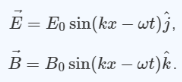# Problem: To understand the formula representing a traveling electromagnetic wave. Light, radiant heat (infrared radiation), X rays, and radio waves are all examples of traveling electromagnetic waves. Electromagnetic waves comprise combinations of electric and magnetic fields that are mutually compatible in the sense that the changes in one generate the other. The simplest form of a traveling electromagnetic wave is a plane wave. For a wave traveling in the x direction whose electric field is in the y direction, the electric and magnetic fields are given byThis wave is linearly polarized in the y direction.What is the wavelength λ of the wave described in the problem introduction?

###### FREE Expert Solution

Wave number, k, is defined as

$\overline{){\mathbf{k}}{\mathbf{=}}\frac{\mathbf{2}\mathbf{\pi }}{\mathbf{\lambda }}}$

In the wave equation, we already have k.###### Problem Details

To understand the formula representing a traveling electromagnetic wave. Light, radiant heat (infrared radiation), X rays, and radio waves are all examples of traveling electromagnetic waves. Electromagnetic waves comprise combinations of electric and magnetic fields that are mutually compatible in the sense that the changes in one generate the other. The simplest form of a traveling electromagnetic wave is a plane wave. For a wave traveling in the x direction whose electric field is in the y direction, the electric and magnetic fields are given byThis wave is linearly polarized in the y direction.

What is the wavelength λ of the wave described in the problem introduction?